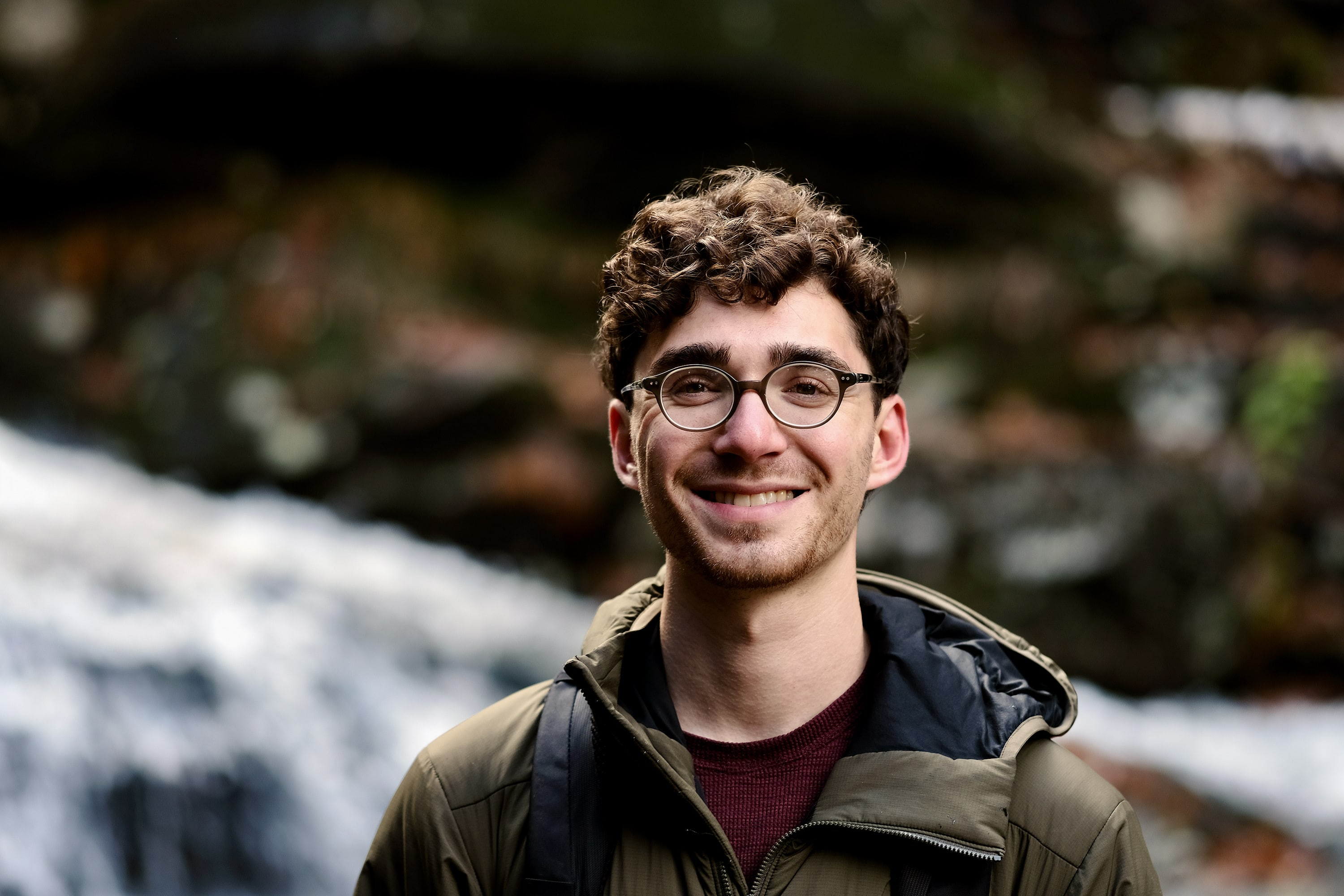# Sam FreedmanOffice: Room 110a, MacMillan Hall
Email: sam_freedman [at] brown [dot] edu

I am a fifth year Ph.D. candidate at Brown.
My research is in flat surfaces and Teichmüller dynamics.
I write SageMath code and contribute to the FlatSurf software suite.
Before Brown, I studied math at the University of Michigan.

Here is my CV.

I am an organizer for the Brown University Math Circle.

I was a TA at the 2021 Summer@ICERM REU on computational billiards.

## Papers

1. Periodic Points on Prym Eigenforms. Preprint.
Abstract: A point of a Veech surface is periodic if it has a finite orbit under the surface's affine automorphism group. We show that the periodic points of Prym eigenforms in the minimal strata of translation surfaces in genera 2, 3 and 4 are the fixed points of the Prym involution. This answers a question of Apisa--Wright and gives a geometric proof of Möller's classification of periodic points of Veech surfaces in the minimal stratum in genus 2.

2. Computing Periodic Points on Veech Surfaces. Preprint. Code.
Abstract: A non-square-tiled Veech surface has finitely many periodic points, i.e. points with finite orbit under the affine automorphism group. We present an algorithm that inputs a non-square-tiled Veech surface and outputs its set of periodic points. Applying our algorithm to Prym eigenforms in the minimal stratum in genus 3, we obtain experimental evidence that these surfaces do not have periodic points, except for the fixed points of the Prym involution.

3. Automorphisms of tropical Hassett spaces. Port. Math. 79 (2022).
Abstract: Given an integer g ≥ 0 and a weight vector w in Q ∩ (0, 1]^n satisfying 2g - 2 + Σ w_i > 0\$, let Δ_{g, w} denote the moduli space of n-marked, w-stable tropical curves of genus g and volume one. We calculate the automorphism group Aut(Δ_{g, w}) for g ≥ 1 and arbitrary w, and we calculate the group Aut(Δ_{0, w}) when w is heavy/light. In both of these cases, we show that Aut(Δ_{g, w}) ≅ Aut(K_w)\$, where K_w is the abstract simplicial complex on {1,…,n} whose faces are subsets with w-weight at most 1. We show that these groups are precisely the finite direct products of symmetric groups. The space Δ_{g, w} may also be identified with the dual complex of the divisor of singular curves in the algebraic Hassett space M‾_{g, w}. Following the work of Massarenti and Mella on the biregular automorphism group Aut(M‾_{g, w}), we show that Aut(Δ_{g, w}) is naturally identified with the subgroup of automorphisms which preserve the divisor of singular curves.

## Travel

• "SaltFlat", University of Utah, January 2023
• "Combinatorics, Dynamics and Geometry on Moduli Spaces", CIRM, September 2022
• Laboratorie de Mathématiques d'Orsay, March-June 2022